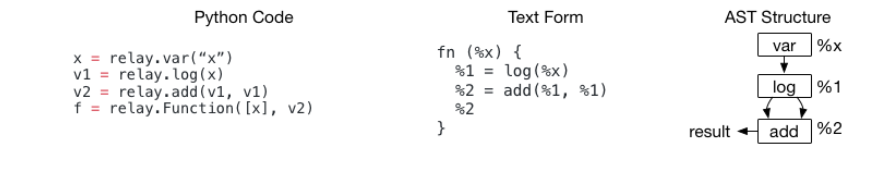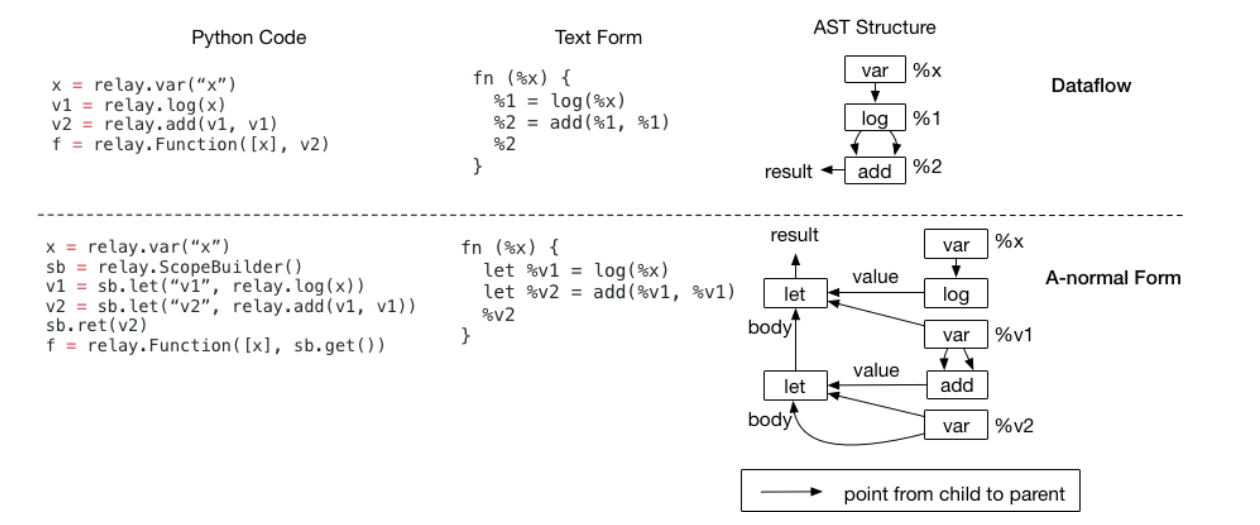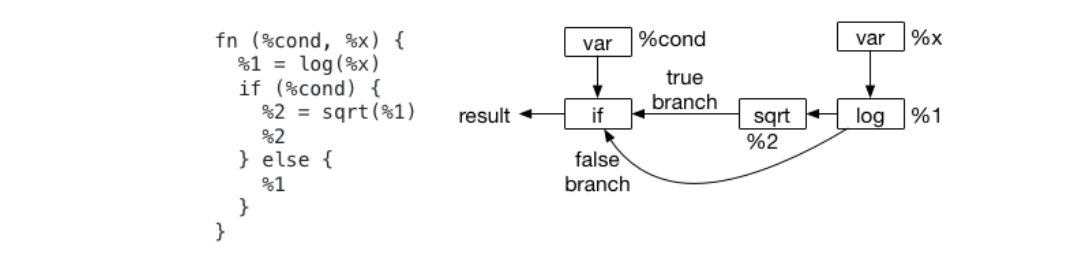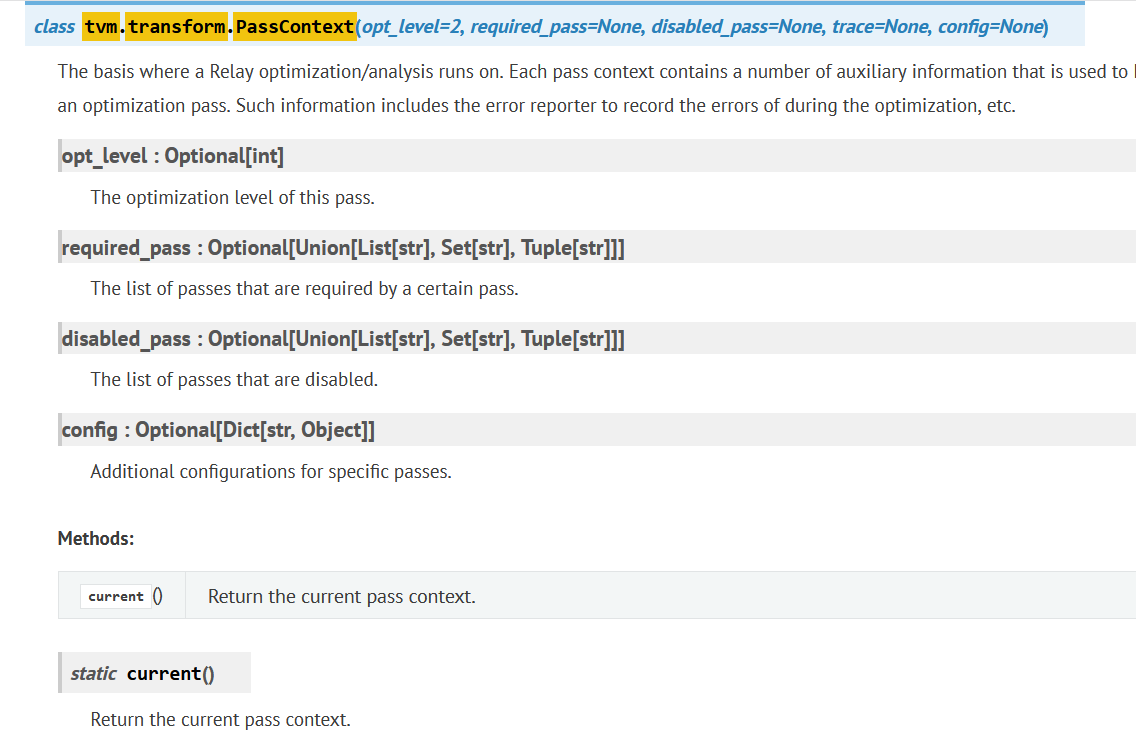【GiantPandaCV导语】这篇文章主要介绍了一下TVM的Relay并介绍了如何基于Relay构建一个Conv+BN+ReLU的小网络，然后介绍了一下TVM中的Pass的工作机制，并较为详细的介绍了RemoveUnusedFunctions，ToBasicBlockNormalForm，EliminateCommonSubexpr三种Pass。其中Relay部分的详细介绍大部分引用自官方文档：https://tvm.apache.org/docs/tutorials/get_started/introduction.html

# 0x0. 介绍¶

######################################################################
# Relay Build
# -----------
# Compile the graph to llvm target with given input specification.
target = "llvm"
target_host = "llvm"
dev = tvm.cpu(0)
with tvm.transform.PassContext(opt_level=3):
lib = relay.build(mod, target=target, target_host=target_host, params=params)


# 0x2. Relay介绍¶

• 支持传统的数据流(DataFlow)风格编程。
• 支持functional-style scoping，并融合了编程语言领域的一些知识，带了一些新的特性（支持Let表达式，支持递归等等）
• 支持数据流风格和函数式风格混合编程。

## 0x2.1 使用Relay建立一个计算图¶• 现有框架通常使用图和子图
• Relay使用函数，例如 – fn（％x），表示图

Relay IR注意到了这个区别。其实深度学习框架用户经常使用这种方式构建计算图，其中经常发生DAG节点重用。然后当我们以文本格式打印Relay程序时，我们每行打印一个CallNode，并为每个CallNode分配一个临时ID(%1, %2)，以便可以在程序的后续部分中引用每个公共节点。

## 0x2.2 Module：支持多个函数（Graphs）¶

def @muladd(%x, %y, %z) {
%1 = mul(%x, %y)
%2
}
def @myfunc(%x) {
%2
}


Module可以被看作Map<GlobalVar, Function>，其中GlobalVar仅仅是一个表示函数名的ID，上面的程序中GlobalVar是@muladd@myfunc。当一个CallNode被用来调用另外一个函数时，相应的GlobalVar被存在CallNode的OP中。它包含了一个间接的等级关系---我们需要使用相应的GlobalVar从Module中查找被调用函数的主体。在这种情况下，我们也可以直接将引用的函数存储为CallNode中的OP。那么为什么需要引入GlobalVar呢？主要原因是为了解耦定义和声明，并支持了函数的递归和延迟声明。

def @myfunc(%x) {
%1 = equal(%x, 1)
if (%1) {
%x
} else {
%2 = sub(%x, 1)
%3 = @myfunc(%2)
%4
}
}


• 有文本形式中间表示，便于开发和 debug
• 支持子图函数、联合模块，便于联合优化
• 前端用户友好，便于调优

## 0x2.3 Let Binding and Scopes¶

Let binding被每一种高级的编程语言应用。在Relay中，他是一个拥有三个字段Let(var, value, body)的数据结构。当我们计算一个Let表达式时，我们首先计算value部分，然后将其绑定到var，最后在body表达式中返回计算结果。## 0x2.4 为什么我们可能需要Let Binding¶

Let Binding的一种关键用法是它可以指定计算的scope。我们看一下下面这个没有使用Let Binding的例子：fn (%x) {
%1 = log(%x)
%2 = fn(%y) {
}
%2
}


Let Binding解决了这些问题，因为值的计算发生在let节点上。在这两个程序中，如果将%1 = log(%x)改成let %v1 = log(%x)，则我们将计算位置明确指定为if scope和闭包之外。可以看到Let Binding为计算端提供了更精确的范围，并且在生成后端代码时会很有用（因为这种范围在IR中）。

## 0x2.5 对IR转换的影响¶

Relay选择同时支持数据流形式和Let Binding。TVM相信让框架开发者选择熟悉的表达形式很重要。但是这确实对我们写通用的Passes产生了一些影响。由于这里还没介绍Passes，以及对Passes理解不深并且我没有使用过Let表达式来构建网络，就不继续介绍具体有哪些影响了。

# 0x3. 基于Relay构建一个自定义的神经网络示例¶

#coding=utf-8
import tvm
from tvm import relay
import numpy as np
from tvm.contrib import graph_executor

# 构造BN
def batch_norm(data,
gamma=None,
beta=None,
moving_mean=None,
moving_var=None,
**kwargs):
name = kwargs.get("name")
kwargs.pop("name")
if not gamma:
gamma = relay.var(name + "_gamma")
if not beta:
beta = relay.var(name + "_beta")
if not moving_mean:
moving_mean = relay.var(name + "_moving_mean")
if not moving_var:
moving_var = relay.var(name + "_moving_var")
return relay.nn.batch_norm(data,
gamma=gamma,
beta=beta,
moving_mean=moving_mean,
moving_var=moving_var,
**kwargs)

# 构造卷积
def conv2d(data, weight=None, **kwargs):
name = kwargs.get("name")
kwargs.pop("name")
if not weight:
weight = relay.var(name + "_weight")
return relay.nn.conv2d(data, weight, **kwargs)

# 构造卷积+BN+ReLU的simpleNet
def simplenet(data, name, channels, kernel_size=(3, 3), strides=(1, 1),
conv = conv2d(
data=data,
channels=channels,
kernel_size=kernel_size,
strides=strides,
data_layout='NCHW',
name=name+'_conv')
bn = batch_norm(data=conv, epsilon=epsilon, name=name + '_bn')
act = relay.nn.relu(data=bn)
return act

data_shape = (1, 3, 224, 224)
kernel_shape = (32, 3, 3, 3)
dtype = "float32"
data = relay.var("data", shape=data_shape, dtype=dtype)
act = simplenet(data, "graph", 32, strides=(2, 2))
func = relay.Function(relay.analysis.free_vars(act), act)

print(func)

np_data = np.random.uniform(-1, 1, (1, 3, 224, 224))

params = {
"graph_conv_weight": tvm.nd.array(np.random.uniform(-1, 1, (32, 3, 3, 3)).astype(dtype)),
"graph_bn_gamma": tvm.nd.array(np.random.uniform(-1, 1, (32)).astype(dtype)),
"graph_bn_beta": tvm.nd.array(np.random.uniform(-1, 1, (32)).astype(dtype)),
"graph_bn_moving_mean": tvm.nd.array(np.random.uniform(-1, 1, (32)).astype(dtype)),
"graph_bn_moving_var": tvm.nd.array(np.random.uniform(-1, 1, (32)).astype(dtype)),
}

with tvm.transform.PassContext(opt_level=3):
lib = relay.build(func, "llvm", params=params)

dev = tvm.cpu(0)
dtype = "float32"
m = graph_executor.GraphModule(lib["default"](dev))
# set inputs
m.set_input("data", tvm.nd.array(np_data.astype(dtype)))
# execute
m.run()
# get outputs
tvm_output = m.get_output(0)


fn (%data: Tensor[(1, 3, 224, 224), float32], %graph_conv_weight, %graph_bn_gamma, %graph_bn_beta, %graph_bn_moving_mean, %graph_bn_moving_var) {
%0 = nn.conv2d(%data, %graph_conv_weight, strides=[2, 2], padding=[1, 1, 1, 1], channels=32, kernel_size=[3, 3]);
%1 = nn.batch_norm(%0, %graph_bn_gamma, %graph_bn_beta, %graph_bn_moving_mean, %graph_bn_moving_var);
%2 = %1.0;
nn.relu(%2)
}


# 0x4. 初识Pass¶

with tvm.transform.PassContext(opt_level=3):
lib = relay.build(func, "llvm", params=params)Pass是TVM中基于Relay IR进行的一系列优化，类似于onnx-simplifier里面用到的onnxoptimizer，它可以简化计算图，去除一些冗余的算子，提高模型的推理效率。TVM将所有的pass都抽象到了tvm/include/tvm/ir/transform.h这个文件中，主要包含PassContext，PassInfo，Pass，以及Sequential。

• RemoveUnusedFunctions

void VisitExpr_(const FunctionNode* func_node) final {
auto func = GetRef<Function>(func_node);
if (visiting_.find(func) == visiting_.end()) {
visiting_.insert(func);
for (auto param : func_node->params) {
ExprVisitor::VisitExpr(param);
}
ExprVisitor::VisitExpr(func_node->body);
}
}

IRModule RemoveUnusedFunctions(const IRModule& module, Array<runtime::String> entry_funcs) {
std::unordered_set<std::string> called_funcs{};
for (auto entry : entry_funcs) {
auto funcs = CallTracer(module).Trace(entry);
called_funcs.insert(funcs.cbegin(), funcs.cend());
}
auto existing_functions = module->functions;
for (auto f : existing_functions) {
auto it = called_funcs.find(f.first->name_hint);
if (it == called_funcs.end()) {
module->Remove(f.first);
}
}
return module;
}


• ToBasicBlockNormalForm

Expr ToBasicBlockNormalFormAux(const Expr& e) {
// calculate all the dependency between nodes.
support::Arena arena;
DependencyGraph dg = DependencyGraph::Create(&arena, e);
/* The scope of the whole expr is global.
* The scope of any subexpr, is the lowest common ancestor of all incoming edge.
* We also record the set of expressions whose scope is lifted.
*/
std::pair<NodeScopeMap, ExprSet> scopes = CalcScope(dg);
return Fill::ToBasicBlockNormalForm(e, dg, &scopes.first, &scopes.second);
}

IRModule ToBasicBlockNormalForm(const IRModule& mod) {
DLOG(INFO) << "ToBBlock:" << std::endl << mod;

auto funcs = mod->functions;
for (const auto& it : funcs) {
ICHECK_EQ(FreeVars(it.second).size(), 0) << "Expected no free variables";
if (const auto* n = it.second.as<FunctionNode>()) {
if (n->GetAttr<String>(attr::kCompiler).defined()) continue;
}
}

for (auto pair : updates) {
}

DLOG(INFO) << "ToBBlock: transformed" << std::endl << mod;

return mod;
}

bool BasicBlockNormalFormCheck(const Expr& e) {
// calculate all the dependency between nodes.
support::Arena arena;
DependencyGraph dg = DependencyGraph::Create(&arena, e);
std::pair<NodeScopeMap, ExprSet> scopes = CalcScope(dg);
for (auto expr : scopes.second) {
LOG(FATAL) << "The expression below violates the basic block normal form in that "
<< "its scope should be lifted:\n"
<< expr;
}
return scopes.second.size() == 0;
}


ToBasicBlockNormalForm这个函数通过遍历Relay IR中的function，将每个function转换为基本块形式（即ToBasicBlockNormalFormAux这个函数），ToBasicBlockNormalFormAux这个函数分成以下几个部分：

• 调用DependencyGraph dg = DependencyGraph::Create(&arena, e)创建一个DependencyGraph，这个数据结构是一个表达式相互依赖的图结构。

• 通过std::pair<NodeScopeMap, ExprSet> scopes = CalcScope(dg)计算每个节点的scope，这个scope可以简单理解为由跳转指令如Ifnode，FunctionNode,LetNode等隔开的那些子图，因为一旦碰到这些节点在上面通过Relay Function创建DependencyGraph就会为这种节点分配一个new_scope标志。然后CalcScope这个函数具体做了哪些事情，我们需要跟进去看一下：

std::pair<NodeScopeMap, ExprSet> CalcScope(const DependencyGraph& dg) {
NodeScopeMap expr_scope;
ExprSet lifted_exprs;
std::unordered_map<DependencyGraph::Node*, Expr> node_to_expr;
// 首先让每个节点都属于一个单独的scope
for (auto expr_node : dg.expr_node) {
node_to_expr[expr_node.second] = expr_node.first;
}
bool global_scope_used = false;
Scope global_scope = std::make_shared<ScopeNode>();
// 使用LCA算法来更新每个节点的真正scope
for (auto it = dg.post_dfs_order.rbegin(); it != dg.post_dfs_order.rend(); ++it) {
DependencyGraph::Node* n = *it;
Scope s;
if (iit == nullptr) {
ICHECK(!global_scope_used);
s = global_scope;
global_scope_used = true;
} else {
s = expr_scope.at(iit->value);
const auto original_s = s;
iit = iit->next;
for (; iit != nullptr; iit = iit->next) {
s = LCA(s, expr_scope.at(iit->value));
}
if (s != original_s && node_to_expr.find(n) != node_to_expr.end()) {
// filter out exprs whose scope do not matter
Expr expr = node_to_expr[n];
if (!expr.as<OpNode>()) {
lifted_exprs.insert(expr);
}
}
}
if (n->new_scope) {
auto child_scope = std::make_shared<ScopeNode>(s);
expr_scope.insert({n, child_scope});
} else {
expr_scope.insert({n, s});
}
}
ICHECK(global_scope_used);
return std::make_pair(expr_scope, lifted_exprs);
}


1. $\text{LCA}({u})=u$

2. $u$$v$ 的祖先，当且仅当 $\text{LCA}(u,v)=u$

3. 如果 $u$ 不为 $v$ 的祖先并且 $v$ 不为 $u$ 的祖先，那么 $u,v$ 分别处于 $\text{LCA}(u,v)$ 的两棵不同子树中；

4. 前序遍历中，$\text{LCA}(S)$ 出现在所有 $S$ 中元素之前，后序遍历中 $\text{LCA}(S)$ 则出现在所有 $S$ 中元素之后；

5. 两点集并的最近公共祖先为两点集分别的最近公共祖先的最近公共祖先，即 $\text{LCA}(A\cup B)=\text{LCA}(\text{LCA}(A), \text{LCA}(B))$

6. 两点的最近公共祖先必定处在树上两点间的最短路上；

7. $d(u,v)=h(u)+h(v)-2h(\text{LCA}(u,v))$，其中 $d$ 是树上两点间的距离，$h$ 代表某点到树根的距离。

/*!
* \file let_list.h
* \brief LetList record let binding and insert let expression implicitly.
*  using it, one can treat AST as value instead of expression,
*  and pass them around freely without fear of AST explosion (or effect duplication).
*  for example, if one write 'b = a + a; c = b + b; d = c + c', the AST will contain 8 'a'.
*  if one instead write 'b = ll.Push(a + a); c = ll.Push(b + b); d = ll.Get(c + c);',
*  the AST will contain 2 'a', as b and c are now variables.


Let_list使得抽象语法树简洁化，不会因为变量的复制导致树的爆炸。具有相同的scope的expr被约束到相同的let_list中，用一个var来表达，这样就将表达式转化为var的形式。一个var也就对应了一个基本块。

• EliminateCommonSubexpr

a = b + c d = b + c

Expr Rewrite_(const CallNode* call, const Expr& post) final {
static auto op_stateful = Op::GetAttrMap<TOpIsStateful>("TOpIsStateful");
Expr new_expr = post;
const CallNode* new_call = new_expr.as<CallNode>();
ICHECK(new_call);
const OpNode* op = new_call->op.as<OpNode>();
StructuralEqual attrs_equal;

if (new_call->args.size() == 0 || op == nullptr || op_stateful.get(GetRef<Op>(op), false)) {
return new_expr;
}
if (fskip_ != nullptr && fskip_(new_expr)) {
return new_expr;
}

auto it = expr_map_.find(new_call->op);
if (it != expr_map_.end()) {
for (const Expr& candidate_expr : it->second) {
if (const CallNode* candidate = candidate_expr.as<CallNode>()) {
bool is_equivalent = true;
// attrs匹配
if (!attrs_equal(new_call->attrs, candidate->attrs)) {
continue;
}
// args匹配
for (size_t i = 0; i < new_call->args.size(); i++) {
if (!new_call->args[i].same_as(candidate->args[i]) &&
!IsEqualScalar(new_call->args[i], candidate->args[i])) {
is_equivalent = false;
break;
}
}
if (!is_equivalent) continue;
return GetRef<Call>(candidate);
}
}
}
expr_map_[new_call->op].push_back(new_expr);
return new_expr;
}


# 0x6. 参考资料¶